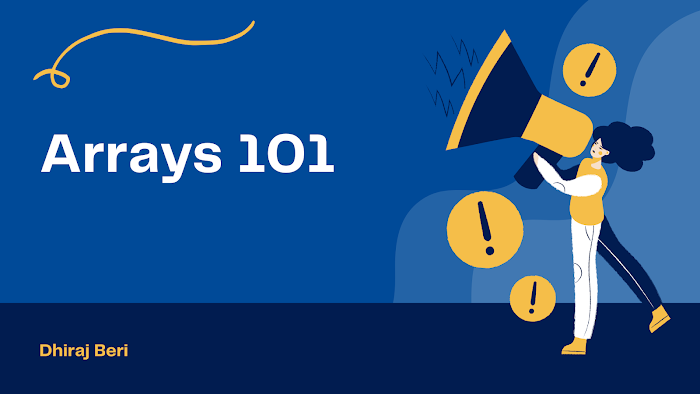# Missing elements in array

You are given a list of n-1 integers and these integers are in the range of 1 to n. There are no duplicates in the list. One of the integers is missing from the list. Write an efficient code to find the missing integer.

Let's take one example
Input: arr[] = {1, 2, 4, 6, 3, 7, 8}
Output: 5
Explanation: The missing number from 1 to 8 is 5

Input: arr[] = {1, 2, 3, 5}
Output: 4
Explanation: The missing number from 1 to 5 is 4

If numbers are natural numbers then it is very easy in that case.
`#include <stdio.h>int main(){    int arr[] = {0, 1, 2, 3, 4, 5, 7};    int sum = 0, i, n = 7;    for (i = 0; i < n; i++)    {        sum = sum + arr[i];    }    int s, missing;    s = n * (n + 1) / 2;    missing = s - sum;    printf("%d", missing);    return 0;}`

Second approach
`#include <stdio.h>int main(){    int arr[] = {3, 4, 5, 7};    int l = arr;    int h = arr;    int n = 4;    int diff = l - 0; //3-0=3    for (int i = 0; i < n; i++)    {        if (arr[i] - i != diff) //3-0=3, 4-1=3, 5-2=3, 7-3=4        {            return i + diff; //3+3 = 6            break;           // we found missing element so break the loop        }    }    return 0;}`

Okay, what if there are multiple missing elements in an array? Seems complex?
`#include <stdio.h>int main(){    int arr[] = {6, 7, 8, 9, 12, 13, 15};    int n = 7;    int l = arr;    int h = arr[n - 1];    int diff = l - 0;    for (int i = 0; i < n; i++)    {        if (arr[i] - i != diff) //naya diff purana diff ke equal nahi hai means element missing hai        {            while (diff < arr[i] - i) //jab tak diff chota hai naye diff ke dab tak loop chalega because ek se jyada element missing hai            {                printf("%d ", i + diff); //missing element                diff++;             }        }    }    return 0;}`

Oops, but in the above method, the time complexity is O(n2) which is not good. Is there is an optimal solution? Let's try it with a hash table. Don't worry if you are not familiar with a hash table, I will introduce it later.
`#include <stdio.h>int main(){    int arr[] = {6, 7, 8, 9, 12, 13, 15};    int n = 7;    int l = arr;    int h = arr[n - 1];    int H[] = {0, 0, 0, 0, 0, 0, 0, 0, 0, 0, 0, 0, 0, 0, 0, 0}; //hash table largest number tak    for (int i = 0; i < n; i++)     {        H[arr[i]]++; //number 0 se 1 ho jayga    }    for (int i = l; i <= h; i++)    {        if (H[i] == 0) //agar abhi bhi koi number 0 hua matlab voh missing element hai        {            printf("%d ", i); //missing element        }    }    return 0;}`

Do you have any other optimal solution for this problem? Try to solve this by yourself and don't focus on the optimal solution if you are a beginner. Just try to solve it by yourself. Good Luck ;)